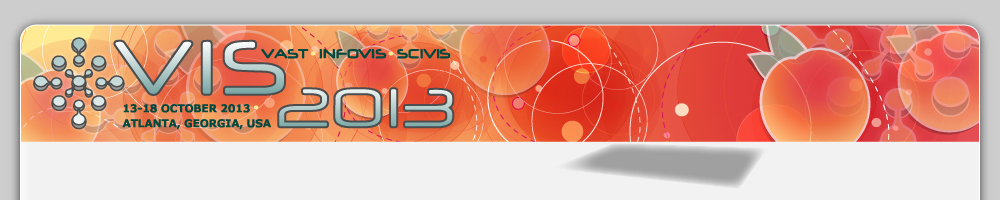13 - 18 OCTOBER 2013, ATLANTA, GEORGIA, USAInterpolation of Non-Gaussian Probability Distributions for Ensemble Visualization

Contributors:
Brad E. Hollister, Dr. Alex Pang
Description
A typical assumption is that ensemble data at each spatial location follows a Gaussian distribution. We investigate the consequences of that assumption when distributions are non-Gaussian. A sufficiently acceptable interpolation scheme needs to be addressed for the interpolation of non-Gaussian distributions. We present two methods to calculate interpolations between two arbitrary distributions and compare them against two baseline methods. The first method uses a Gaussian Mixture Model (GMM) to represent distributions. The second method is a non-parametric approach that interpolates between quantiles in the cumulative distribution functions. The baseline methods for comparison purposes are: (a) using a Gaussian representation and interpolating the means and standard deviations, and (b) forming a new distribution based on the interpolation of individual realizations of the ensemble. We show that the two proposed non-Gaussian interpolation methods have the following behavior: the interpolated distributions do not decompose to more constituent Gaussian distributions than the highest modality of those being interpolated, and do not have variances less than the smallest variance from the grid points being interpolated. Finally,we compare these four interpolation methods when used in the analysis of scalar and vector fields of ensemble data sets, particularly in areas where the distribution is non-Gaussian.